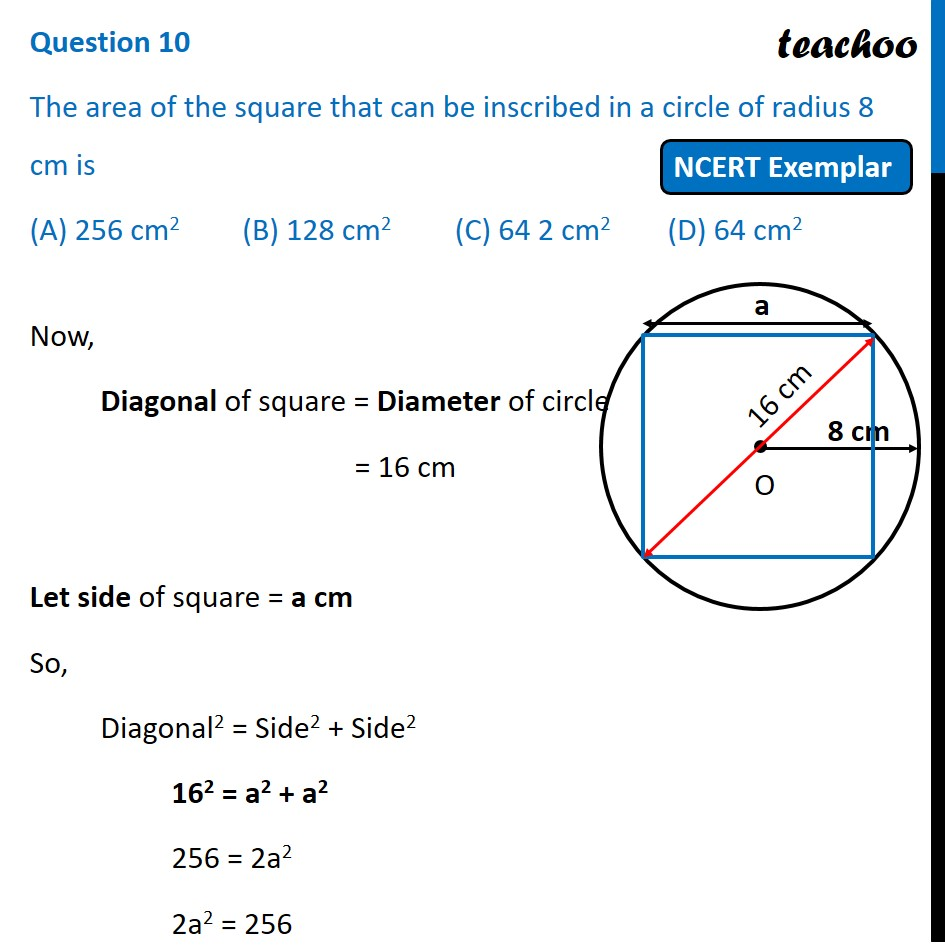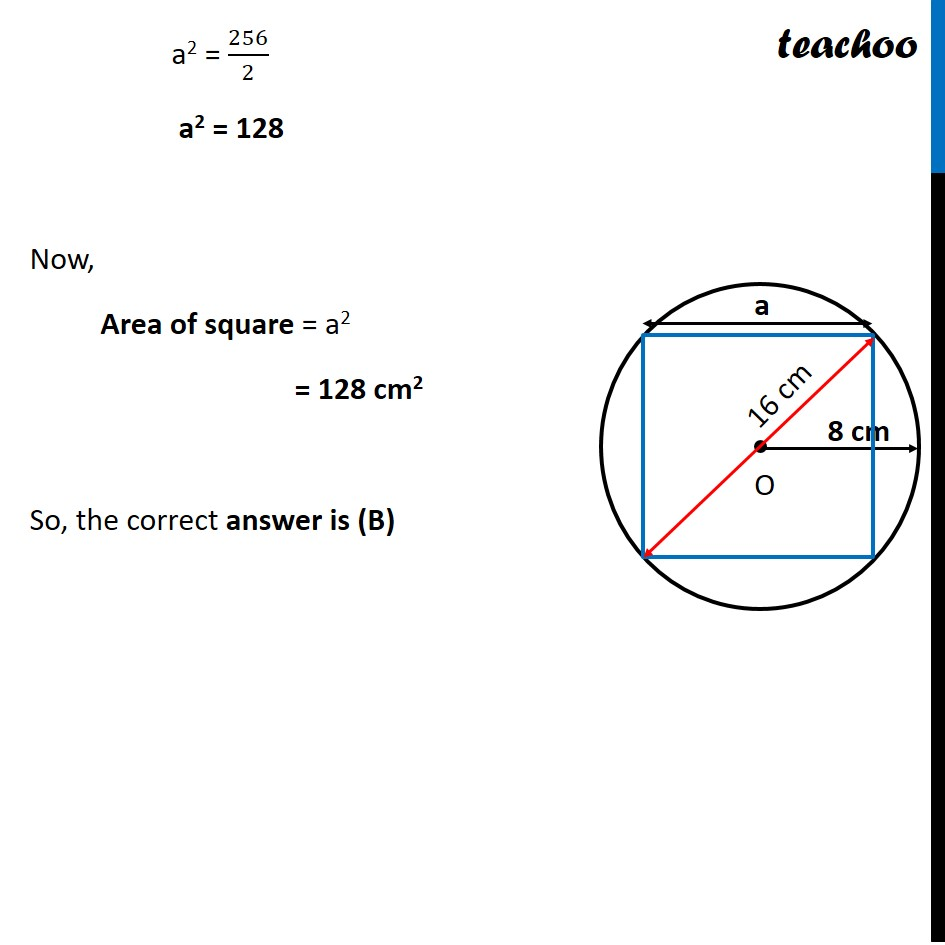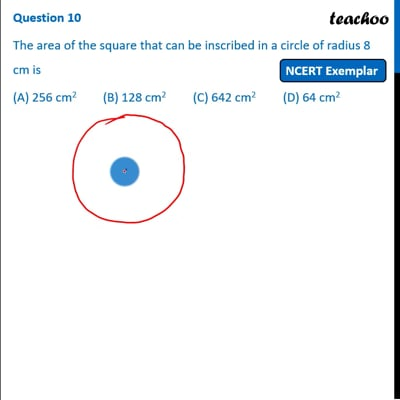NCERT Exemplar - MCQ

Chapter 11 Class 10 Areas related to Circles
Serial order wise

## (D) 64 cm 2This video is only available for Teachoo black users

Learn in your speed, with individual attention - Teachoo Maths 1-on-1 Class

### Transcript

Question 10 The area of the square that can be inscribed in a circle of radius 8 cm is (A) 256 cm2 (B) 128 cm2 (C) 64 2 cm2 (D) 64 cm2 Now, Diagonal of square = Diameter of circle = 16 cm Let side of square = a cm So, Diagonal2 = Side2 + Side2 162 = a2 + a2 256 = 2a2 2a2 = 256 a2 = 256/2 a2 = 128 Now, Area of square = a2 = 128 cm2 So, the correct answer is (B) a2 = 256/2 a2 = 128 Now, Area of square = a2 = 128 cm2 So, the correct answer is (B)